# Forms of Retrieval Sequential Retrieval TwoStep Retrieval Retrieval

• Slides: 10Forms of Retrieval • Sequential Retrieval • Two-Step Retrieval • Retrieval with Indexed Cases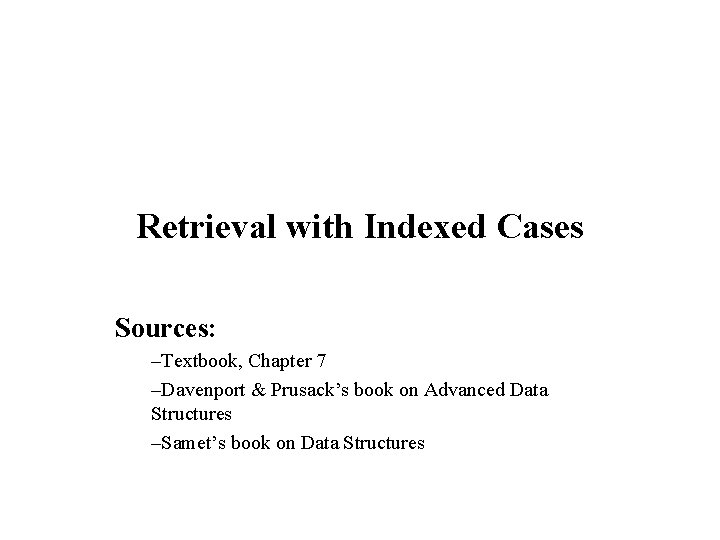Retrieval with Indexed Cases Sources: –Textbook, Chapter 7 –Davenport & Prusack’s book on Advanced Data Structures –Samet’s book on Data Structures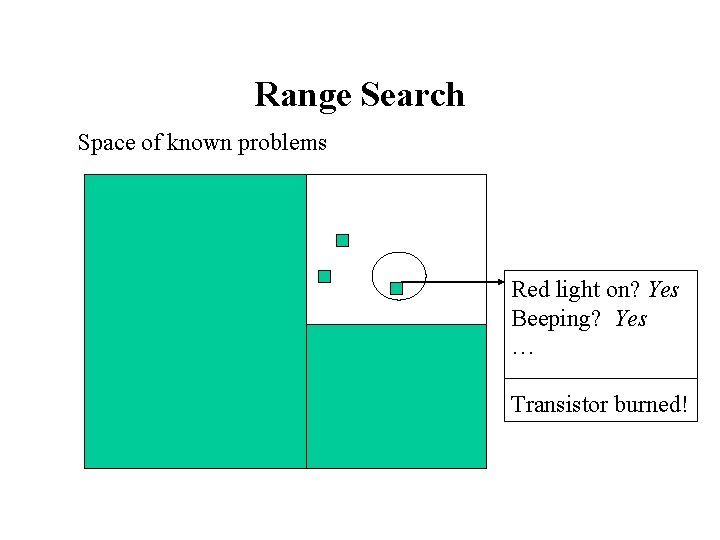Range Search Space of known problems Red light on? Yes Beeping? Yes … Transistor burned!k-d Trees • Idea: Partition of the case base in smaller fragments • Representation of a k-dimensional space in a binary tree • Similar to a decision tree: comparison with nodes • During retrieval: ØSearch for a leaf, but ØUnlike decision trees backtracking may occurDefinition: k-d Trees • Given: ØK types: T 1, …, Tk for the attributes A 1, …, Ak ØA case base CB containing cases in T 1 … Tk ØA parameter b (size of bucket) • A K-D tree T(CB) for a case base CB is a binary tree defined as follows: ØIf |CB| < b then T(CB) is a leaf node (a bucket) ØElse T(CB) defines a tree such that: §The root is marked with an attribute Ai and a value v in Ai and §The 2 k-d trees T({c CB: c. i-attribute < v}) and T({c CB: c. i-attribute v}) are the left and right subtrees of the root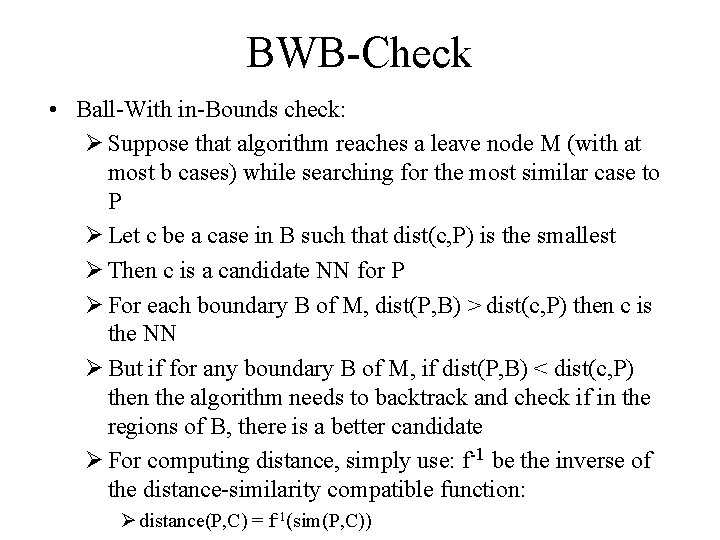BWB-Check • Ball-With in-Bounds check: Ø Suppose that algorithm reaches a leave node M (with at most b cases) while searching for the most similar case to P Ø Let c be a case in B such that dist(c, P) is the smallest Ø Then c is a candidate NN for P Ø For each boundary B of M, dist(P, B) > dist(c, P) then c is the NN Ø But if for any boundary B of M, if dist(P, B) < dist(c, P) then the algorithm needs to backtrack and check if in the regions of B, there is a better candidate Ø For computing distance, simply use: f-1 be the inverse of the distance-similarity compatible function: Ø distance(P, C) = f-1(sim(P, C))BOB-Check • Ball-Out of-Bounds check: Ø Used during backtracking Ø Checks if for the boundary B defined in the node: dist(P, B) < dist(c, P) q. Where c is our current candidate for best case (e. g. , the closest case to P in the initial bucket) Ø If the condition is true, The algorithm needs to check if in those boundary’s regions, there is a better candidate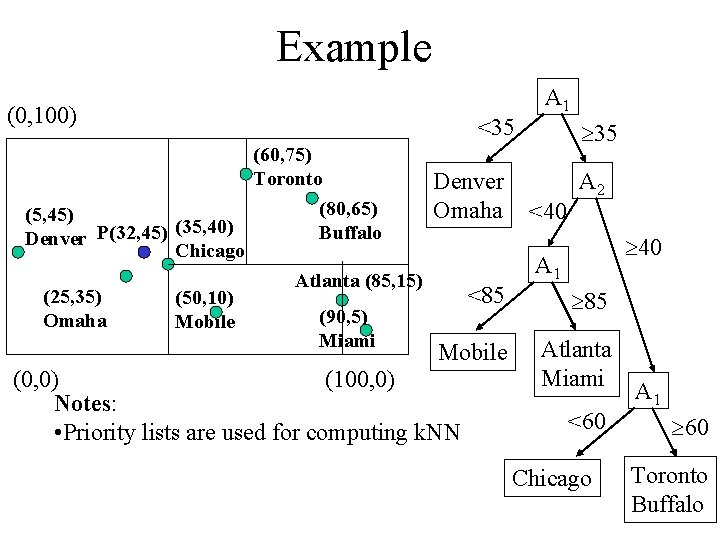Example (0, 100) (60, 75) Toronto (80, 65) (5, 45) (35, 40) Buffalo Denver P(32, 45) Chicago Atlanta (85, 15) (25, 35) (50, 10) (90, 5) Omaha Mobile Miami <35 Denver Omaha <85 Mobile (0, 0) (100, 0) Notes: • Priority lists are used for computing k. NN A 1 35 <40 A 2 40 A 1 85 Atlanta Miami <60 Chicago A 1 60 Toronto Buffalo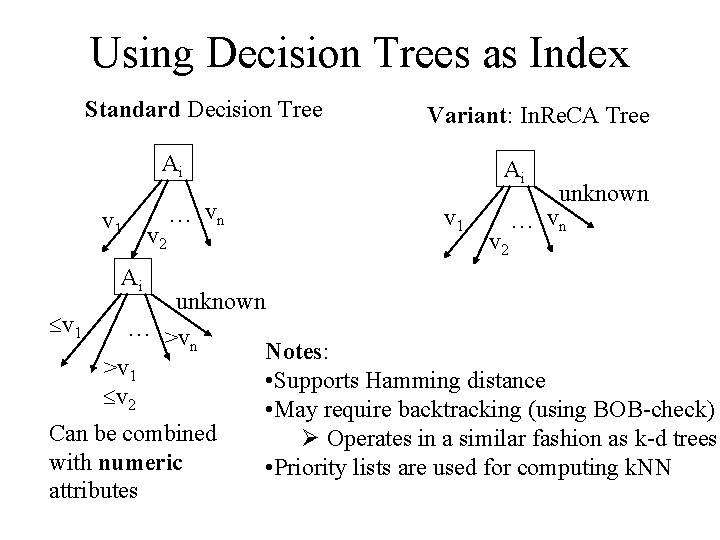Using Decision Trees as Index Standard Decision Tree Variant: In. Re. CA Tree Ai v 1 Ai v 2 … vn Ai v 1 v 2 unknown … vn unknown v 1 … >v n Notes: >v 1 • Supports Hamming distance v 2 • May require backtracking (using BOB-check) Can be combined Ø Operates in a similar fashion as k-d trees with numeric • Priority lists are used for computing k. NN attributes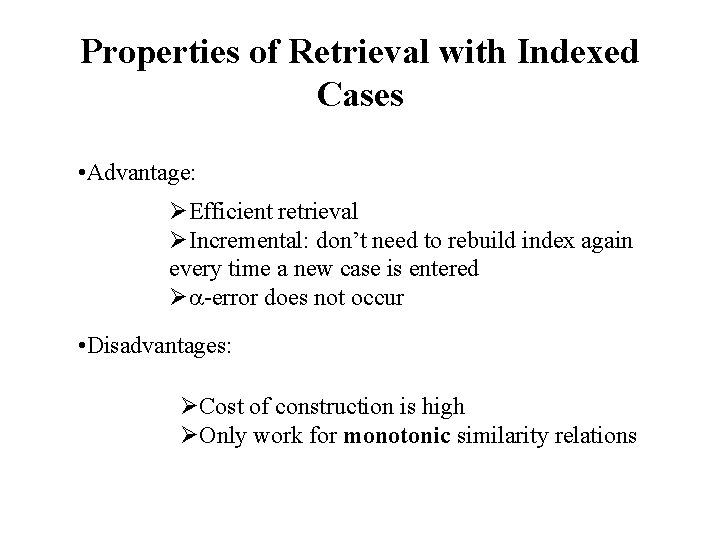Properties of Retrieval with Indexed Cases • Advantage: ØEfficient retrieval ØIncremental: don’t need to rebuild index again every time a new case is entered Ø -error does not occur • Disadvantages: ØCost of construction is high ØOnly work for monotonic similarity relations